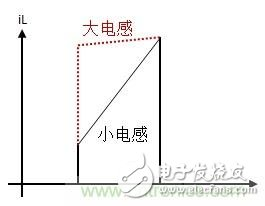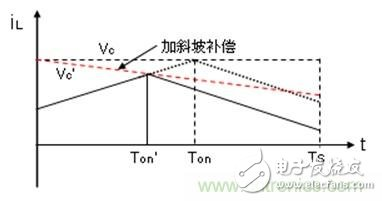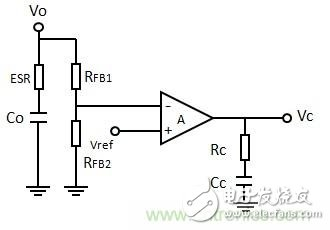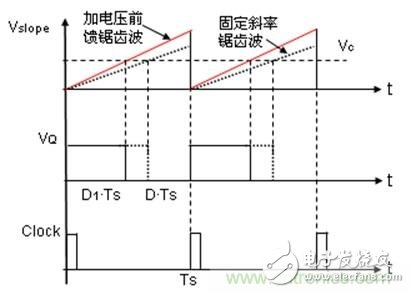# How to switch the switching power supply system voltage mode and current mode?

Usually when discussing these two modes of operation, the ideal voltage mode and current mode are referred to. However, in practical applications, the current mode switching power supply system, when the output load changes, or in some operating conditions, adds some compensation signals for the stability of the system. At this time, the system introduces part of the current mode. Voltage mode characteristics, or full voltage mode.

1.1 Current mode tends to voltage mode at light load

When the power system enters light load or no load, the converter usually operates in burst mode and skip mode. For the skip mode, the converter enters the discontinuous current mode, and the turn-on time of the high-side switch is the minimum on-time set by the controller. At the same time, there are some switching cycles, and the high-side switch is not conducting, that is, shielding. , or skip some switching pulses to maintain the output voltage regulation.

Note that at light load or no load, the current signal is small, and the system is difficult to detect the current signal. On the other hand, since the turn-on time of the high-side switch is fixed to the minimum on-time, it is not affected by the current detection signal. The adjustment, the current feedback does not actually work, and does not participate in the feedback link. The system now operates in a standard voltage mode.

For burst mode, the output voltage is completely controlled by the hysteresis comparator. The hysteresis comparator control sets the output voltage within the allowable upper and lower limits by detecting changes in the output voltage. The system is also operating at standard. Voltage mode.

1.2 Using large inductance values â€‹â€‹tends to voltage mode

The selection and design of the output inductor is based on the steady state and transient requirements of the output DC voltage. Larger inductor values â€‹â€‹reduce the output ripple current and ripple voltage and reduce core losses, but the time to change the inductor current during load transients increases, while increasing the cost and size of the inductor. Smaller inductance values â€‹â€‹result in lower DC copper losses, but AC core losses and AC winding resistance losses become larger.Figure 1: Different inductor currents

When a large inductor is used at the same time, the slope of the inductor current is reduced. In an ideal state, if the inductor value is infinite, the inductor current is a DC value throughout the switching period, and the current detection signal is not active, that is, the standard Voltage mode. Therefore, the larger the inductance value used, the closer the control of the current mode is to the voltage mode, and the worse the dynamic characteristics of the system during load transients. Therefore, for current mode, the compromise is to choose the peak-to-peak value of the inductor ripple current between 20% and 40% of the output load current rating.

1.3 Current mode of slope compensation tends to voltage mode

In theory, when the duty cycle is greater than 50%, the current mode is added with slope compensation, and the system can work stably. Otherwise, subharmonic oscillations will occur. In practical applications, when the duty cycle is greater than 40%, slope compensation is added. When the duty ratio is greater than 50%, the slope compensation, because the inductance is fully excited, and the demagnetization is insufficient, the output voltage will be higher than the preset value and will continue to rise until the slower voltage control loop adjusts the current setting. Until the fixed point, then the output voltage drops again below the expected value, forming a subharmonic oscillation.

The typical characteristic of subharmonic oscillation is that in one switching cycle, the pulse width is wide, and in the next switching cycle, the pulse width is narrowed, and the pulse width is widened every three switching cycles, and so on. At this point, you can see that the output voltage is unstable, and sometimes you can hear the noise of the audio.

In Figure 2, the red line slope compensation, the solid triangle wave is the current waveform of the inductor with slope compensation, and the dotted line is the current waveform of the inductor without slope compensation. If the sawtooth voltage of the falling edge is used, it is applied to the output of the voltage error amplifier to control the current detection signal; if the sawtooth voltage of the rising edge is used, it is applied to the current detection signal, and then with the voltage error amplifier The output is compared.

Note that the internal slope compensation will reduce the total current ramp, ie the slope compensation will reduce the slope of the true inductor current, which can remove the disturbance of the average inductor current with different duty cycles, so that the peak inductor current is controlled. Finally, it converges on the average inductor current. Therefore, the composite waveform signal is composed of a slope compensation signal and an actual inductor current signal, which causes the converter to convert from the current mode to the voltage mode.

The larger the slope compensation is, the closer the converter is to the voltage mode. When the slope of the applied compensation ramp signal is increased to a certain extent, the peak current mode control is converted into voltage mode control. Because the slope compensation signal is completely replaced by the triangular wave of the oscillating circuit, it becomes the voltage mode control, but the current signal at this time can be regarded as a current feedforward signal.Figure 2: Slope Compensation

The slope compensation also reduces the gain of the current loop, reducing the current limit set in the system, and reducing the actual load current value of the system.

When in the no-load state, the output current is zero and the slope compensation signal amplitude is relatively large, the peak current mode control actually becomes the voltage mode control.

Usually when discussing these two modes of operation, the ideal voltage mode and current mode are referred to. However, in practical applications, the voltage mode switching power supply system, that is, the system feedback loop does not introduce a current sampling signal, but other methods are used to introduce a certain degree of current feedback, and the voltage mode shifts to the current mode, thereby improving the system. Dynamic ringing.

2.1 Average current mode formed by sampling the output capacitor ESR in voltage mode

The ideal voltage mode is difficult to work stably over the entire voltage input range and output load variation under certain feedback network parameters. Output load variation can be optimized by increasing the output capacitance while using a capacitor with a large ESR value, although this results in increased system cost and volume while increasing the output voltage ripple.

Generally speaking, it is intuitively understood that the output capacitor ESR and the output capacitor form a zero point. For the current mode, this zero point is not necessary because the current mode is a single-order system, and this zero point causes the gain of the high frequency to increase, and the system is susceptible to high Frequency noise interference. So the current mode or the use of ESR very low ceramic capacitors, so that the ESR zero point is raised to a higher frequency, will not affect the feedback system, or add a pole to offset the role of zero in the high frequency band, the method of adding poles is On the ITH (Vc) pin and a capacitor to ground.Figure 3: Output Capacitor ESR

The voltage mode is a second-order system formed by LC. The introduction of this zero point can offset a pole of the LC double pole to a certain extent, making it convert to a single-order system. The larger the ESR, the more obvious the effect. Therefore, the voltage mode output voltage usually uses a large ESR capacitor.

On the other hand, note that the output voltage is:

Vo=Vco+ESR*DIL

DIL=a*Io

Vco is the voltage on the capacitive reactance of the output capacitor, DIL is the ripple current of the inductor, and a is the current ripple coefficient, which is generally 0.2-0.4.

The small signal value of the output voltage is:

DVo=VDco+D(ESR*a*Io)

If the ESR is small, the latter one can be ignored. However, since the voltage mode usually uses an output capacitor with a large ESR value, the ESR cannot be ignored. Because of the ESR, it is equivalent to the feedback signal of the input voltage. A certain degree of current mode is introduced, and the current mode feedback amount is: D (ESR*a*Io).

The ESR of the output capacitor sends the sampled current signal to the input of the voltage error amplifier, and the output voltage signal is added together, amplified by the voltage error amplifier, and sent to the PWM comparator. The working principle is equivalent to the average current feedback. In the voltage mode, the use of ESR's large output capacitor is equivalent to introducing a certain degree of average current mode, thereby increasing the dynamic response of the system to the output load change and improving the stability of the system.

2.2 Input voltage feedforward current mode in voltage mode

For input voltage variations, input voltage feedforward techniques are currently commonly used to increase the system's response to input voltage changes. In the voltage mode, the slope of the sawtooth wave generated by the internal clock signal is fixed at k, as indicated by the dashed line in Figure 2. In the absence of voltage feedforward, the resulting duty cycle is D*Ts, which has the following formula:

Vc=k*D*Ts

The input voltage feedforward is to add a slope that varies with the input voltage to the internal sawtooth wave, or subtract this slope from the Vc signal. If the input voltage feedforward is applied to the internal sawtooth wave, if the slope of the applied feedforward voltage ramp is ks, the sum of the internal sawtooth wave and the applied slope is: k+ks.

The slope of the feedforward pre-pressure increases as the input voltage increases. ks is proportional to Vin, ie: ks is proportional to kVin*Vin, so the duty cycle at this time is:

D1=Vc/(k+ks)*Ts=Vc/(k+kVin*Vin)*Ts

The duty cycle decreases as the input voltage increases. As indicated by the solid line in Figure 2, the system responds to changes in the input voltage in advance.Figure 4: Feed mode before voltage mode is added to the input voltage

If efficiency is not considered, the power balance can be obtained: Vin*Iin=Vo*Io, so there are:

Ks=kVin*Vo*Io/Iin

As can be seen from the above equation, the applied input voltage feedforward signal is also the input current signal. In fact, it can be understood that the input voltage feedforward technique is to superimpose a certain current feedback in an ideal voltage mode to form a certain current feedback, thereby increasing the response of the system to input voltage changes.

Household Electrical Appliances

tcl , https://www.tclgroupss.com